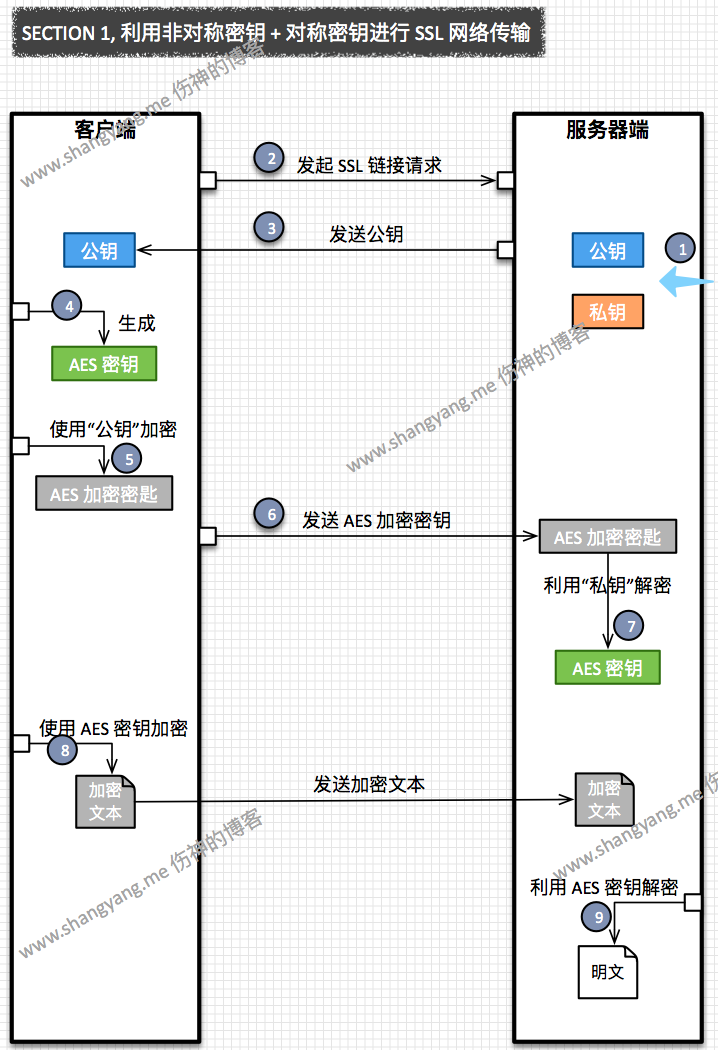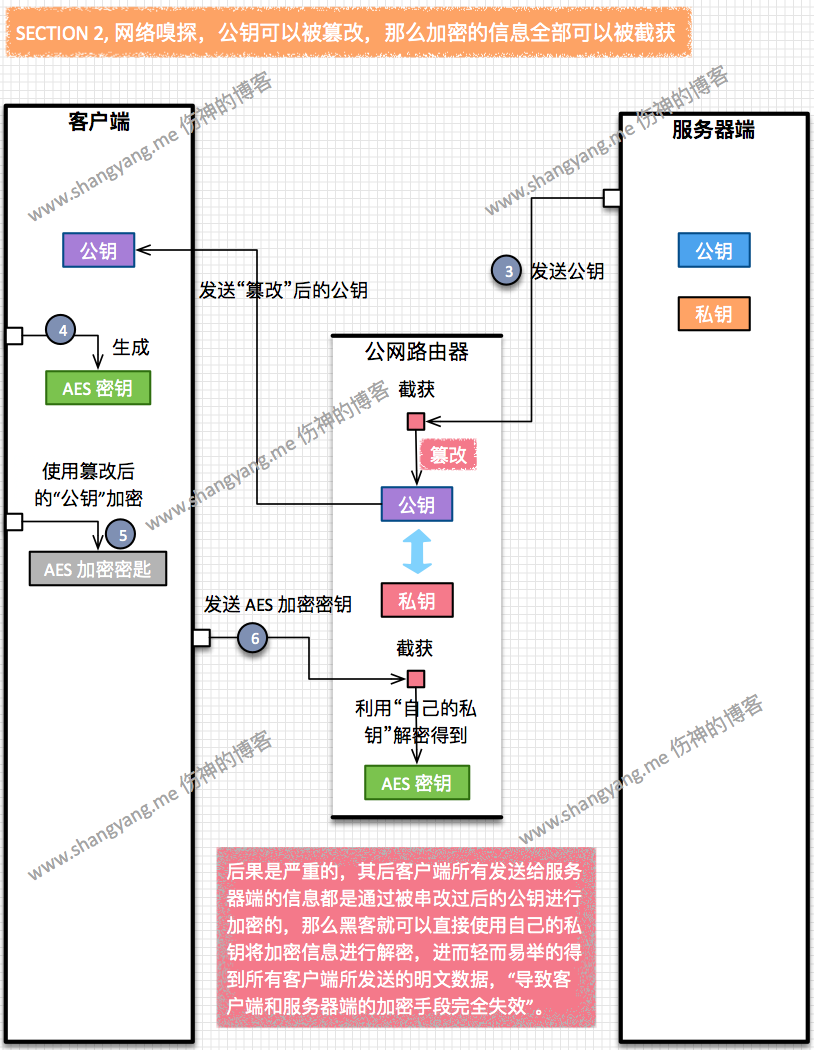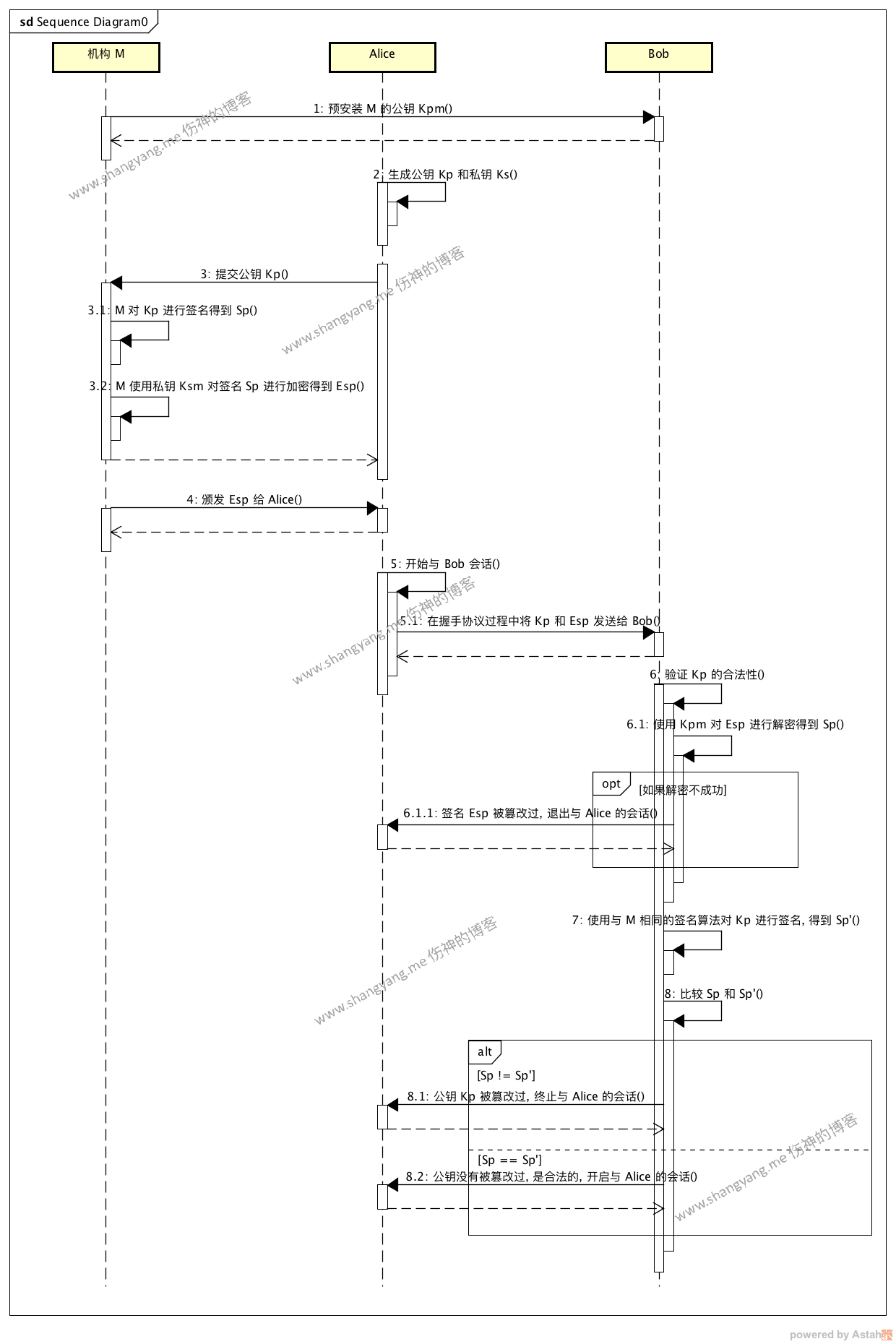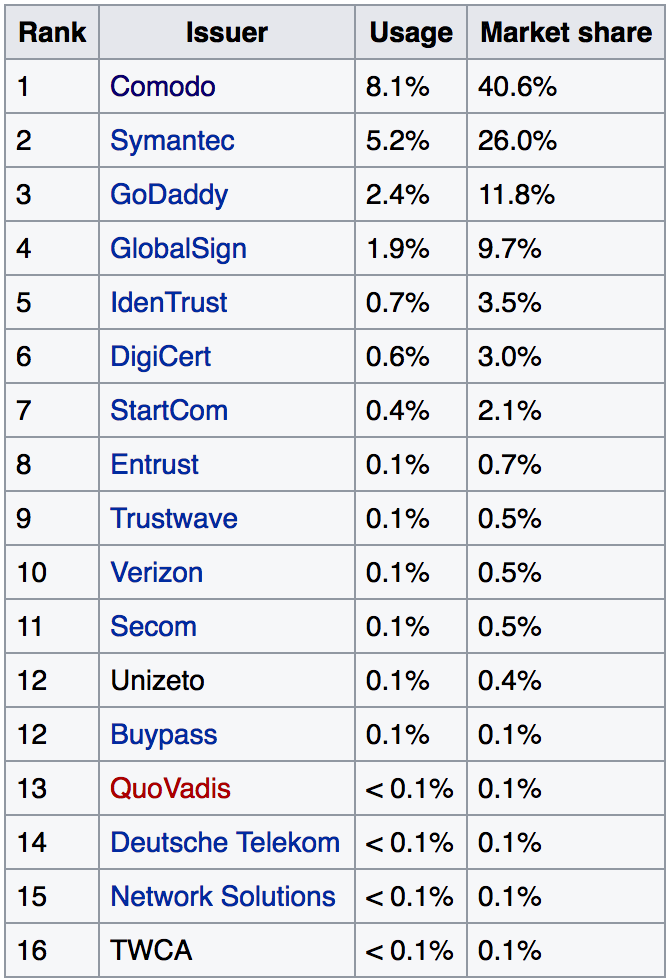# 公钥被篡改# PKI

PKI 的核心职责就是通过一些列的措施保证公钥的合法性，那么它是如何保证公钥不被篡改，是合法的呢？

## 如何保证公钥不被篡改的

1. Alice 将自己的公钥 $K_{p}$ 提交给 $M$；
2. $M$ 使用签名算法，比如 MD5，对 Alice 的原生公钥 $K_p$ 进行签名得到 $S_p$；
3. 然后再通过 $K_{s(m)}$ 对 $S_p$ 进行加密得到加密后的签名 $E_{sp}$；
4. 最后将 $E_{sp}$ 颁发给 Alice；

Alice 如何将公钥发送给 Bob？

• Alice 将加密后的签名 $E_{sp}$ 和公钥 $K_p$ 一起发送给 Bob；
备注，其实签名 $E_{sp}$ 和公钥 $K_p$ 被纳入一张证书(Certificate)中，Alice 发送给 Bob 的其实就是这么一张证书；但是，整整 X.509 证书包含的内容比这个复杂许多，不过概念上是等价的；这里，也就是证书的由来了。不仅要知其然，更要知其所以然..

Bob 如何保证接收到的 Alice 的公钥没有被篡改过？

Bob 拿到 Alice 的证书以后，做如下操作，(为了区分，这里将传输过程中 Alice 的公钥命名为 $K_p’$)

1. 通过 $M$ 的公钥 $K_{p(m)}$ 对 $E_{sp}$ 进行解密，得到 $S_p$；
注意，如果 $E_{sp}$ 被篡改了，这里是无法解密得到 $S_p$，也就是说，要保证解密成功，$E_{sp}$ 一定是没有被篡改过的；
2. 然后使用双方所约定好的签名算法，比如 MD5，对 $K_p’$ 进行签名，得到 $S_p’$
3. 最后比较 $S_p$ 与 $S_p’$ 是否相等，若相等，则表示发送过程中的公钥 $K_p’$ 没有被篡改过，否则，则可以断言，$K_p’$ 被篡改过，于是整个通讯不安全、不可靠；

（备注，这里的机构 $M$ 有个行业用语叫做 $CA$ 既是 Certification Authority；）

## 概念流程图• Step 6.1.1: 断定签名 $E_{sp}$ 被篡改过；
要能够断定这种情况，保证 $E_{sp}$ 一定没有被篡改过，需要公钥基础设施(既 PKI )的保证，保证签名颁发机构 $M$ 的公钥是无法被篡改的

• Step 8.1: $S_p != S_p’$，则可以断定公钥是被篡改过得；
这里面更深层次的原因是，通过公钥认证机构得到的签名 $S_{p}$ 与 Bob 自己得到的公钥签名 $S_{p}$ 不匹配，则可断定，Alice 的公钥 $K_p$ 被篡改过；

## KPI 包含哪些元素

1. CA (Certificate Authority)，证书认证机构
对公钥、用户身份信息、域名等信息进行签名，生成相关的电子证书；并将电子证书颁发给申请用户；
另外还会通过其专有的协议来判断证书是否有效（是否超过使用有效期）,如果证书失效，将会生成证书回收列表既是 certificate revocation list ，该部分内容涉及到 Authority revocation lists 的相关内容，从 https://en.wikipedia.org/wiki/Certificate_authority 摘要其核心内容如下，

An authority revocation list (ARL) is a form of certificate revocation list (CRL) containing certificates issued to certificate authorities, contrary to CRLs which contain revoked end-entity certificates.

https://tools.ietf.org/html/rfc5280#section-4.1.2.6 标注中也描述了有关 CRL 协议和标准；

2. RA (Registration Authority)，注册认证机构
看下 https://en.wikipedia.org/wiki/Public_key_infrastructure 中的描述，

A registration authority which verifies the identity of entities requesting their digital certificates to be stored at the CA

是用来验证证书申请机构的身份的；不过现在 CA 和 RA 并没有完全区分，往往两者表示同一个角色；
用户需要像该机构提交一张 CSR 格式的证书(该证书的后缀名为 .csr)，既是 certificate signing request 来申请，里面需要填写诸如你的域名，公司名，部门名称，城市名，地区名，国家名，电子邮件等等证明你身份的信息；

### CA 机构# Reference

Public key infrastructure: https://en.wikipedia.org/wiki/Public_key_infrastructure
Certificate Authority(CA): https://en.wikipedia.org/wiki/Certificate_authority